Anzeige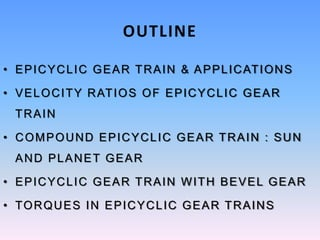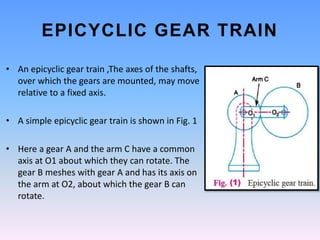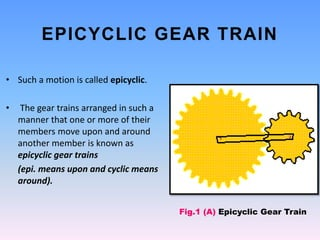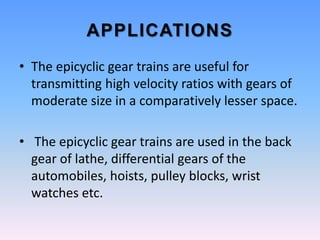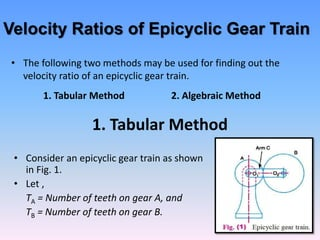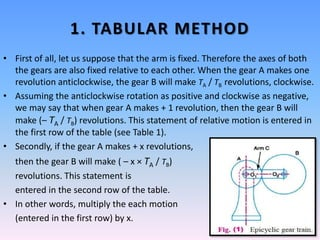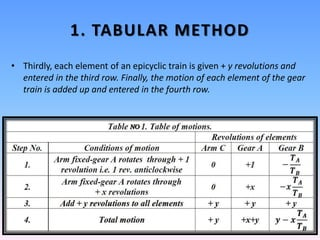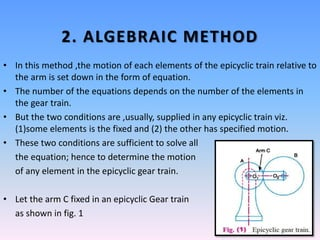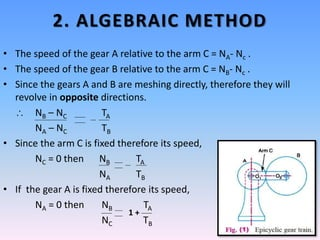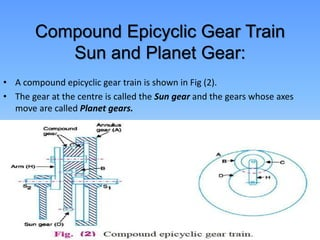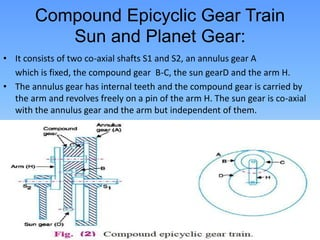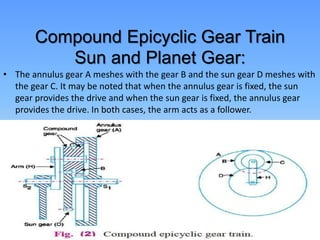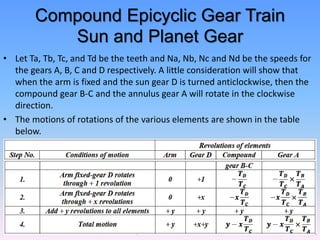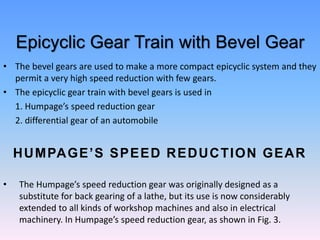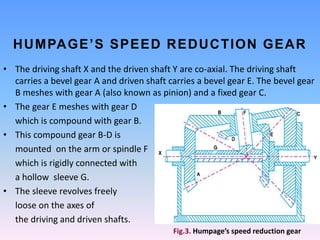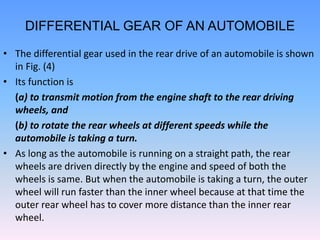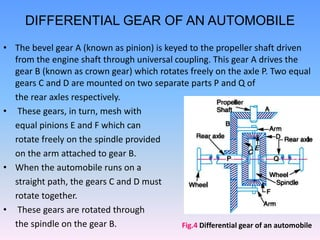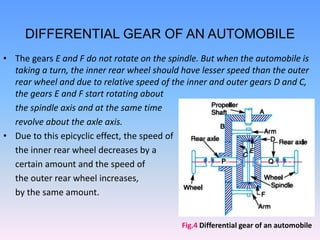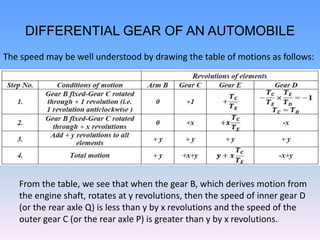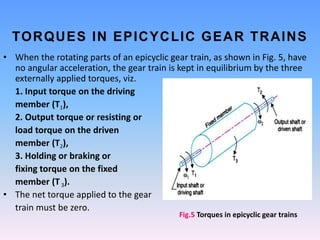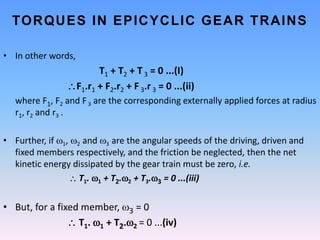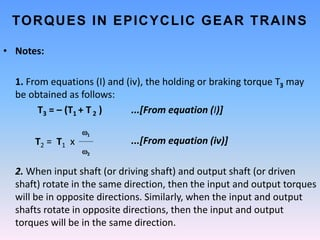1 von 24
Anzeige

### A presentation On Epicycle Gear Train

1. A Presentation on Epicycle Gear Train Manthan Thakkar B.Tech(RE&EE) College of Renewable Energy & Environmental Engineering
2. OUTLINE • EPICYCLIC GEAR TRAIN & APPLICATIONS • VELOCITY RATIOS OF EPICYCLIC GEAR TRAIN • COMPOUND EPICYCLIC GEAR TRAIN : SUN AND PLANET GEAR • EPICYCLIC GEAR TRAIN WITH BEVEL GEAR • TORQUES IN EPICYCLIC GEAR TRAINS
3. EPICYCLIC GEAR TRAIN • An epicyclic gear train ,The axes of the shafts, over which the gears are mounted, may move relative to a fixed axis. • A simple epicyclic gear train is shown in Fig. 1 • Here a gear A and the arm C have a common axis at O1 about which they can rotate. The gear B meshes with gear A and has its axis on the arm at O2, about which the gear B can rotate.
4. • Such a motion is called epicyclic. • The gear trains arranged in such a manner that one or more of their members move upon and around another member is known as epicyclic gear trains (epi. means upon and cyclic means around). Fig.1 (A) Epicyclic Gear Train EPICYCLIC GEAR TRAIN
5. APPLICATIONS • The epicyclic gear trains are useful for transmitting high velocity ratios with gears of moderate size in a comparatively lesser space. • The epicyclic gear trains are used in the back gear of lathe, differential gears of the automobiles, hoists, pulley blocks, wrist watches etc.
6. Velocity Ratios of Epicyclic Gear Train • The following two methods may be used for finding out the velocity ratio of an epicyclic gear train. 1. Tabular Method 2. Algebraic Method 1. Tabular Method • Consider an epicyclic gear train as shown in Fig. 1. • Let , TA = Number of teeth on gear A, and TB = Number of teeth on gear B.
7. 1. TABULAR METHOD • First of all, let us suppose that the arm is fixed. Therefore the axes of both the gears are also fixed relative to each other. When the gear A makes one revolution anticlockwise, the gear B will make TA / TB revolutions, clockwise. • Assuming the anticlockwise rotation as positive and clockwise as negative, we may say that when gear A makes + 1 revolution, then the gear B will make (– TA / TB) revolutions. This statement of relative motion is entered in the first row of the table (see Table 1). • Secondly, if the gear A makes + x revolutions, then the gear B will make ( – x × TA / TB) revolutions. This statement is entered in the second row of the table. • In other words, multiply the each motion (entered in the first row) by x.
8. 1. TABULAR METHOD • Thirdly, each element of an epicyclic train is given + y revolutions and entered in the third row. Finally, the motion of each element of the gear train is added up and entered in the fourth row.
9. 2. ALGEBRAIC METHOD • In this method ,the motion of each elements of the epicyclic train relative to the arm is set down in the form of equation. • The number of the equations depends on the number of the elements in the gear train. • But the two conditions are ,usually, supplied in any epicyclic train viz. (1)some elements is the fixed and (2) the other has specified motion. • These two conditions are sufficient to solve all the equation; hence to determine the motion of any element in the epicyclic gear train. • Let the arm C fixed in an epicyclic Gear train as shown in fig. 1
10. 2. ALGEBRAIC METHOD • The speed of the gear A relative to the arm C = NA- Nc . • The speed of the gear B relative to the arm C = NB- Nc . • Since the gears A and B are meshing directly, therefore they will revolve in opposite directions.  NB – NC TA NA – NC TB • Since the arm C is fixed therefore its speed, NC = 0 then NB TA NA TB • If the gear A is fixed therefore its speed, NA = 0 then NB TA NC TB 1 +
11. Compound Epicyclic Gear Train Sun and Planet Gear: • A compound epicyclic gear train is shown in Fig (2). • The gear at the centre is called the Sun gear and the gears whose axes move are called Planet gears.
12. Compound Epicyclic Gear Train Sun and Planet Gear: • It consists of two co-axial shafts S1 and S2, an annulus gear A which is fixed, the compound gear B-C, the sun gearD and the arm H. • The annulus gear has internal teeth and the compound gear is carried by the arm and revolves freely on a pin of the arm H. The sun gear is co-axial with the annulus gear and the arm but independent of them.
13. Compound Epicyclic Gear Train Sun and Planet Gear: • The annulus gear A meshes with the gear B and the sun gear D meshes with the gear C. It may be noted that when the annulus gear is fixed, the sun gear provides the drive and when the sun gear is fixed, the annulus gear provides the drive. In both cases, the arm acts as a follower.
14. Compound Epicyclic Gear Train Sun and Planet Gear • Let Ta, Tb, Tc, and Td be the teeth and Na, Nb, Nc and Nd be the speeds for the gears A, B, C and D respectively. A little consideration will show that when the arm is fixed and the sun gear D is turned anticlockwise, then the compound gear B-C and the annulus gear A will rotate in the clockwise direction. • The motions of rotations of the various elements are shown in the table below.
15. Epicyclic Gear Train with Bevel Gear • The bevel gears are used to make a more compact epicyclic system and they permit a very high speed reduction with few gears. • The epicyclic gear train with bevel gears is used in 1. Humpage’s speed reduction gear 2. differential gear of an automobile HUMPAGE’S SPEED REDUCTION GEAR • The Humpage’s speed reduction gear was originally designed as a substitute for back gearing of a lathe, but its use is now considerably extended to all kinds of workshop machines and also in electrical machinery. In Humpage’s speed reduction gear, as shown in Fig. 3.
16. HUMPAGE’S SPEED REDUCTION GEAR • The driving shaft X and the driven shaft Y are co-axial. The driving shaft carries a bevel gear A and driven shaft carries a bevel gear E. The bevel gear B meshes with gear A (also known as pinion) and a fixed gear C. • The gear E meshes with gear D which is compound with gear B. • This compound gear B-D is mounted on the arm or spindle F which is rigidly connected with a hollow sleeve G. • The sleeve revolves freely loose on the axes of the driving and driven shafts. Fig.3. Humpage’s speed reduction gear
17. DIFFERENTIAL GEAR OF AN AUTOMOBILE • The differential gear used in the rear drive of an automobile is shown in Fig. (4) • Its function is (a) to transmit motion from the engine shaft to the rear driving wheels, and (b) to rotate the rear wheels at different speeds while the automobile is taking a turn. • As long as the automobile is running on a straight path, the rear wheels are driven directly by the engine and speed of both the wheels is same. But when the automobile is taking a turn, the outer wheel will run faster than the inner wheel because at that time the outer rear wheel has to cover more distance than the inner rear wheel.
18. • The bevel gear A (known as pinion) is keyed to the propeller shaft driven from the engine shaft through universal coupling. This gear A drives the gear B (known as crown gear) which rotates freely on the axle P. Two equal gears C and D are mounted on two separate parts P and Q of the rear axles respectively. • These gears, in turn, mesh with equal pinions E and F which can rotate freely on the spindle provided on the arm attached to gear B. • When the automobile runs on a straight path, the gears C and D must rotate together. • These gears are rotated through the spindle on the gear B. Fig.4 Differential gear of an automobile DIFFERENTIAL GEAR OF AN AUTOMOBILE
19. • The gears E and F do not rotate on the spindle. But when the automobile is taking a turn, the inner rear wheel should have lesser speed than the outer rear wheel and due to relative speed of the inner and outer gears D and C, the gears E and F start rotating about the spindle axis and at the same time revolve about the axle axis. • Due to this epicyclic effect, the speed of the inner rear wheel decreases by a certain amount and the speed of the outer rear wheel increases, by the same amount. Fig.4 Differential gear of an automobile DIFFERENTIAL GEAR OF AN AUTOMOBILE
20. DIFFERENTIAL GEAR OF AN AUTOMOBILE The speed may be well understood by drawing the table of motions as follows: From the table, we see that when the gear B, which derives motion from the engine shaft, rotates at y revolutions, then the speed of inner gear D (or the rear axle Q) is less than y by x revolutions and the speed of the outer gear C (or the rear axle P) is greater than y by x revolutions.
21. TORQUES IN EPICYCLIC GEAR TRAINS • When the rotating parts of an epicyclic gear train, as shown in Fig. 5, have no angular acceleration, the gear train is kept in equilibrium by the three externally applied torques, viz. 1. Input torque on the driving member (T1), 2. Output torque or resisting or load torque on the driven member (T2), 3. Holding or braking or fixing torque on the fixed member (T3). • The net torque applied to the gear train must be zero. Fig.5 Torques in epicyclic gear trains
22. • In other words, T1 + T2 + T 3 = 0 ...(I) F1.r1 + F2.r2 + F 3.r 3 = 0 ...(ii) where F1, F2 and F3 are the corresponding externally applied forces at radius r1, r2 and r3 . • Further, if 1, 2 and 3 are the angular speeds of the driving, driven and fixed members respectively, and the friction be neglected, then the net kinetic energy dissipated by the gear train must be zero, i.e.  T1. 1 + T2.2 + T3.3 = 0 ...(iii) • But, for a fixed member, 3 = 0  T1. 1 + T2.2 = 0 ...(iv) TORQUES IN EPICYCLIC GEAR TRAINS
23. • Notes: 1. From equations (I) and (iv), the holding or braking torque T3 may be obtained as follows: T3 = – (T1 + T 2 ) ...[From equation (I)] ...[From equation (iv)] 2. When input shaft (or driving shaft) and output shaft (or driven shaft) rotate in the same direction, then the input and output torques will be in opposite directions. Similarly, when the input and output shafts rotate in opposite directions, then the input and output torques will be in the same direction. T2 = T1 x 1 2 TORQUES IN EPICYCLIC GEAR TRAINS
Anzeige